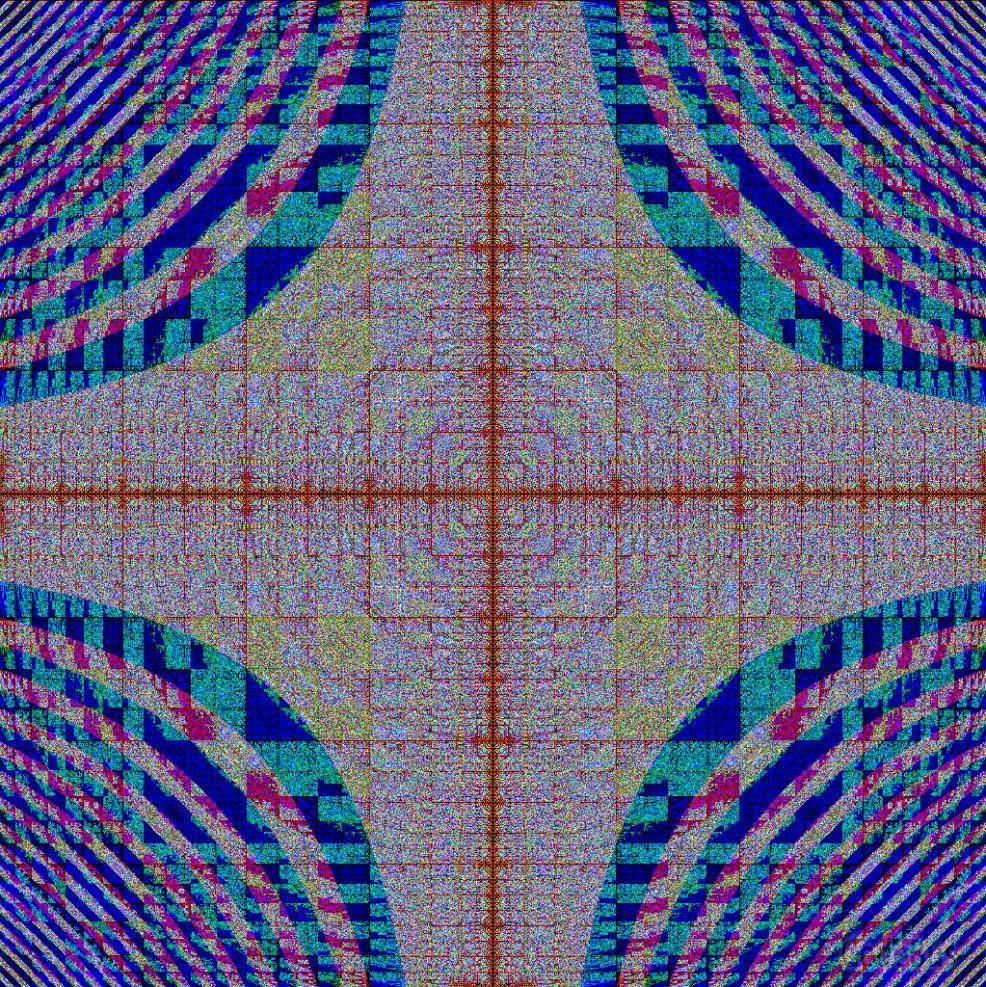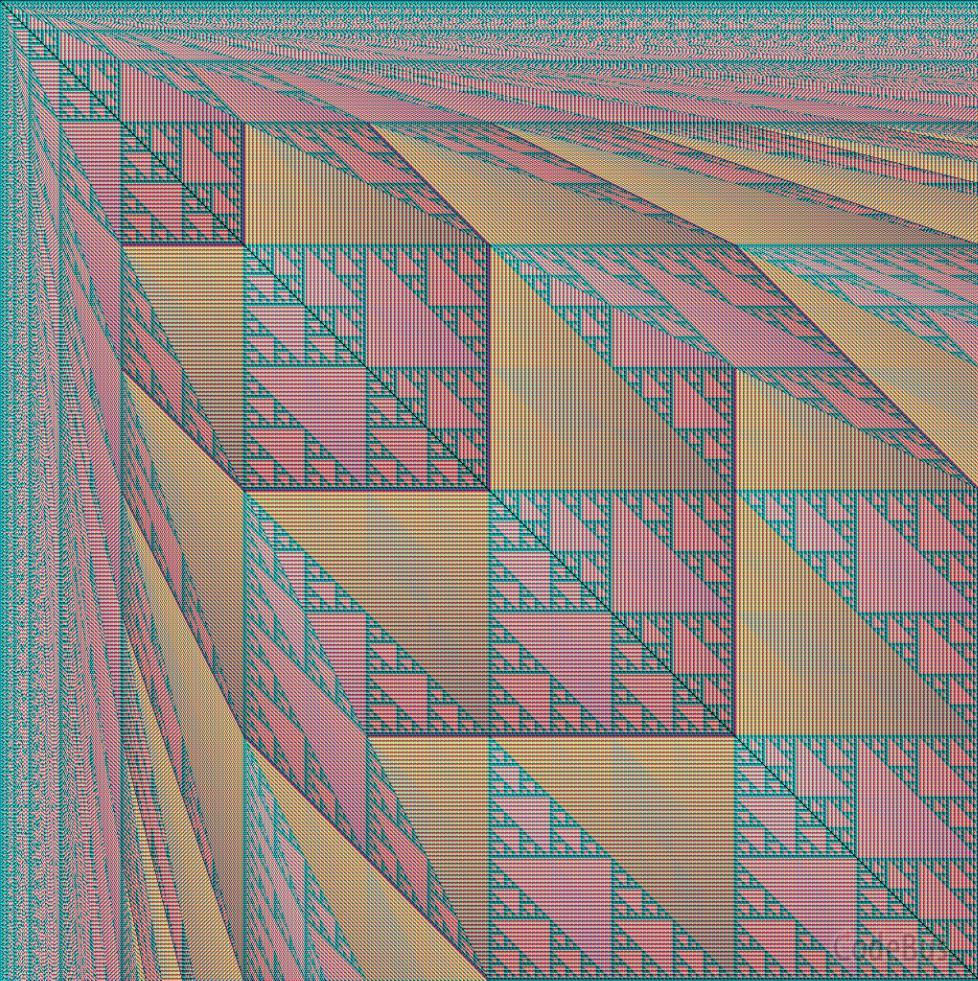# 比赛简介

``````// 红色
unsigned char RD(int i, int j)
{
}

// 绿色
unsigned char GR(int i, int j)
{
}

// 蓝色
unsigned char BL(int i, int j)
{
}
``````

``````// 程序名称：Tweetable Mathematical Art 比赛的 EasyX 版本
// 原比赛地址：https://codegolf.stackexchange.com/questions/35569/tweetable-mathematical-art
//
#include <graphics.h>
#include <math.h>
#include <stdlib.h>
#include <time.h>

#define DIM		1024
#define DM1		(DIM-1)
#define _sq(x)	((x)*(x))							// 平方
#define _cb(x)	abs((x)*(x)*(x))					// 立方的绝对值
#define _cr(x)	(unsigned char)(pow((x),1.0/3.0))	// 立方根（运算结果取整）

unsigned char GR(int,int);

unsigned char BL(int,int);

unsigned char RD(int i, int j)
{
}

unsigned char GR(int i, int j)
{
}

unsigned char BL(int i, int j)
{
}

int main()
{
initgraph(1024, 1024);
srand((unsigned)time(NULL));

DWORD *p = GetImageBuffer(NULL);

for (int y = 0; y < 1024; y++)
for (int x = 0; x < 1024; x++)
//			putpixel(x, y, RGB(RD(x, y), GR(x, y), BL(x, y)));					// 逐步显示（慢）
p[(y << 10) + x] = RD(x, y) << 16 | GR(x, y) << 8 | BL(x, y);		// 直接写显示缓冲区（快）

FlushBatchDraw();

getmessage(EX_CHAR);	// 按任意键退出
closegraph();
return 0;
}
``````

# 示例

``````unsigned char RD(int i,int j)
{
unsigned short n = (unsigned short)sqrt((double)(_sq(i-DIM/2)*_sq(j-DIM/2))*2.0);
n &= DM1;
return (n >> 8 | n << 8) * 255 / 1023;
}

unsigned char GR(int i,int j)
{
unsigned short n = (unsigned short)sqrt((double)(
(_sq(i-DIM/2)|_sq(j-DIM/2))*
(_sq(i-DIM/2)&_sq(j-DIM/2))
));
n &= DM1;
return (n >> 8 | n << 8) * 255 / 1023;
}

unsigned char BL(int i,int j)
{
unsigned short n = (unsigned short)sqrt((double)(_sq(i-DIM/2)&_sq(j-DIM/2))*2.0);
n &= DM1;
return (n >> 8 | n << 8) * 255 / 1023;
}
````````````unsigned char RD(int i,int j)
{
unsigned short n = i&&j?(i%j)&(j%i):0;
n &= DM1;
return (n >> 8 | n << 8) * 255 / 1023;
}

unsigned char GR(int i,int j)
{
unsigned short n = i&&j?(i%j)+(j%i):0;
n &= DM1;
return (n >> 8 | n << 8) * 255 / 1023;
}

unsigned char BL(int i,int j)
{
unsigned short n = i&&j?(i%j)|(j%i):0;
n &= DM1;
return (n >> 8 | n << 8) * 255 / 1023;
}
``````（更新中）

# 附注：关于原比赛的一点 bug

``````// NOTE: compile with g++ filename.cpp -std=c++11
//    also, if this doesn't work for you, try the alternate char-based version

#include <iostream>
#include <cmath>
#define DIM 1024
#define DM1 (DIM-1)
#define _sq(x)	((x)*(x))							// square
#define _cb(x)	abs((x)*(x)*(x))					// absolute value of cube
#define _cr(x)	(unsigned short)(pow((x),1.0/3.0))	// cube root

unsigned short GR(int,int);
unsigned short BL(int,int);

unsigned short RD(int i,int j){
}
unsigned short GR(int i,int j){
}
unsigned short BL(int i,int j){
}

void pixel_write(int,int);
FILE *fp;
int main(){
fp = fopen("MathPic","wb");
fprintf(fp, "P6\n%d %d\n1023\n", DIM, DIM);
for(int j=0;j<DIM;j++)
for(int i=0;i<DIM;i++)
pixel_write(i,j);
fclose(fp);
return 0;
}
void pixel_write(int i, int j){
static unsigned short color;
color = RD(i,j)&DM1;
color = GR(i,j)&DM1;
color = BL(i,j)&DM1;
fwrite(color, 2, 3, fp);
}
``````

• 如果在 Windows 下生成 ppm（P6 编码）并在 Windows 下查看，无法得出指定效果。
• 如果在 Linux 下生成 ppm（P6 编码）并在 Linux 下查看，无法得出指定效果。
• 如果在 Windows 下生成 ppm（P3 编码，ASCII 格式）并在 Linux 下查看，无法得出指定效果。

``````unsigned char RD(int i,int j)
{
return i&&j?(i%j)&(j%i):0;
}
``````

``````unsigned char RD(int i,int j)
{
unsigned short n = i&&j?(i%j)&(j%i):0;
n &= DM1;
return (n >> 8 | n << 8) * 255 / 1023;
//	return n * 255 / 1023;					// 有些参赛选手的代码在 Linux 下编译，无需交换高低位，就用这行代码替代上一行
}
``````